Courses

# Test: Hydraulic Machines Level - 1

## 20 Questions MCQ Test Engineering Mechanics | Test: Hydraulic Machines Level - 1

Description
This mock test of Test: Hydraulic Machines Level - 1 for Civil Engineering (CE) helps you for every Civil Engineering (CE) entrance exam. This contains 20 Multiple Choice Questions for Civil Engineering (CE) Test: Hydraulic Machines Level - 1 (mcq) to study with solutions a complete question bank. The solved questions answers in this Test: Hydraulic Machines Level - 1 quiz give you a good mix of easy questions and tough questions. Civil Engineering (CE) students definitely take this Test: Hydraulic Machines Level - 1 exercise for a better result in the exam. You can find other Test: Hydraulic Machines Level - 1 extra questions, long questions & short questions for Civil Engineering (CE) on EduRev as well by searching above.
QUESTION: 1

### Multi stage centrifugal pumps are used for

Solution: A multi stage centrifugal pump does not increase the discharge, it increases the head (pressure).

QUESTION: 2

### A Pelton wheel turbine operates under a head of 125 m at a speed of 2000 rpm. To operate very close to the maximum efficiency, the mean diameter of the Pelton wheel is nearly

Solution: Assuming ideal nozzle

Vjet = √2 × g × 12.5

= 49.5 m⁄s

For maximum η bucket speed (u) must be

u = 1 / 2 × Vjet = 24.76

Also u = πdN / 60

Substituting values we get

d = 0.236 m ≈ 24 cm

QUESTION: 3

### If D is the diameter of impeller at inlet, w is the width of impeller at inlet and Vf is the velocity of flow at inlet, then discharge through a centrifugal pump is equal to

Solution: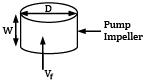Q = πDwVf

QUESTION: 4

A jet of water issues from a nozzle with a velocity 20 m ⁄ s on a flat plate moving away from it at 10 m ⁄ s. The cross-sectional area of the jet is 0.01 m2 and the density of water = 1000 kg⁄m3. The force developed on the plate is

Solution: F = ρQ(Vjet − u)

= ρ × Areajet × (Vjet − u)2

= 1000 × 0.01 × (20 − 10)2

F = 1000 N

QUESTION: 5

A Francis turbine running at 200 rpm develops a power of 5000 kW under a head of 25 m. The power output under a head of 100 m will be

Solution: For a Francis turbine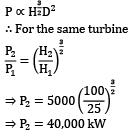QUESTION: 6

A centrifugal compressor is suitable for which of the following?

Solution: Centrifugal compressors can’t give high pressure ratio like reciprocating compressors but their discharge is higher than reciprocating compressors.

QUESTION: 7

A turbine develops 500 kW power under a head of 100 m at 200 rpm. What would be its speed under a head of 81 m?

Solution: Given

P1 = 500 kW

h1 = 100 m

N1 = 200 rpm

N2 =?

h2 = 81 m

We know H ∝ N2D2

For same turbine H ∝ N2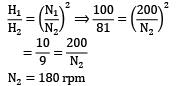QUESTION: 8

Match List-I with List-II and select the correct answer

List-I List-II

a. Pelton wheel (single jet) 1. Medium discharge, low head

b. Francis Turbine 2. High discharge, low head

c. Kaplan Turbine 3. Medium discharge, medium head

Codes: a b c

Solution: Pelton is a high head, low discharge turbine.

Francis is a medium head, medium discharge turbine.

Kaplan is a low head, high discharge turbine.

QUESTION: 9

Pick up the incorrect statement for centrifugal pumps.

Solution: Discharge α (speed) × (diameter)3 so (A) is wrong Head ∝ (speed)2 × (diameter)2

QUESTION: 10

Formation and collapse of vapour bubbles are believed to be the root cause for cavitation in hydraulic turbines. Most favorable condition for the formation of bubbles is set in the turbines at

Solution: The entrance of the draft tube experiences the lowest pressure in a reaction turbine, so the chances of cavitation are the highest out there, as local pressure may drop below vapour pressure.

QUESTION: 11

A pump is defined as a device which converts

Solution: A pump is a device that consumes mechanical energy and converts it into hydraulic energy, mainly pressure head.

QUESTION: 12

The correct sequence of the centrifugal pump components through which the fluid flow is

Solution: The strainer is used to filter out the solid debris from entering the pump; hence it is installed at the mouth of the suction pipe. Then the water flows through the suction pipe and into the impeller and then it is delivered to the outlet through the delivery pipe.

QUESTION: 13

For a reciprocating water pump having cylinder diameter d and crank radius r, if W is the weight of water lifted, the coefficient of discharge is

Solution: The coefficient of discharge = Actual discharge/theoretical discharge, The theoretical weight of fluid delivered = ρgπd2r⁄2 where πd2r⁄2 is the volume swept by the piston. The actual weight of fluid delivered = W.

QUESTION: 14

Cavitation parameter is defined by

Solution: Cavitation parameter is given by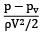where p → Absolute pressure pv → Vapour pressure corresponding to local temperature V → Velocity of flowing fluid ρ → Density of flowing fluid

QUESTION: 15

Both pressure and velocity of steam drops in the moving blades of

Solution: Don’t be confused by the words like steam turbine or compounding. Impulse turbine can only utilize the impulse (kinetic) head and not the reaction (pressure) head. So the drop in pressure can only occur in a reaction turbine.

QUESTION: 16

A pump running at 1000 rpm consumes 1 kW and generates a head of 10 m of water. When it is operated at 2000 rpm, its power consumption and head generated would be

Solution: As the pump diameter is constant, by similarities law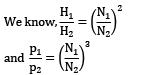Substituting the values of

N1 = 1000 rpm p1 = 1 kW

H1 = 10 m

N2 = 2000 rpm gives us

H2 = 40 m and p2 = 8 kW

QUESTION: 17

Which one of the following is impulse turbine?

Solution: Pelton wheel is an impulse turbine, Francis and Kaplan are reaction turbines.

QUESTION: 18

The specific speed of a turbine is the speed of an imaginary turbine, identical with the given turbine, which

Solution: Specific speed is the speed of a geometrically similar turbine which produces unit power under the unit head.

QUESTION: 19

The specific speed of a pump is defined as the speed of the unit of such a size that it

Solution: Specific speed of pump is such a speed at which a geometrically similar pump will produce unit discharge at unit head.

QUESTION: 20

Consider the following statements:

1. The wheel can be operated freely in air

2. Pressure at the exit of the nozzle is atmospheric

3. Pressure does not vary along the moving vanes

4. Change in direction of momentum imparts thrust over moving vanes

Which of the above statements are applied to impulse turbine?

Solution: As impulse turbines only use the impulse (kinetic) head of liquid, there is no change in pressure; hence the pressure is same everywhere inside the impulse turbine and equal to atmospheric. So 1, 2 and 3 are correct. Now the force acting on the wheel is due to the change of momentum. And since liquids are incompressible and the wheel is open to the atmosphere, the inlet and outlet velocity of water must be constant (ignoring frictional losses) to conserve the mass flow rate. So the change in momentum is only because of its change in direction and not magnitude. So, all the statements are correct.